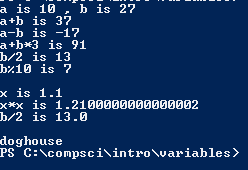# Assignment #17 MoreMathematicalOperations

## Code

```        /// Name: Lacey Reese
/// Period: 5
/// Program Name: MoreMathematicalOperations
/// File Name: MoreMathematicalOperations.java
/// Date Finished 9/23/2015

public class MoreMathematicalOperations
{
public static void main( String[] args )
{
int a, b, c, d, e, f, g;
double x, y, z;
String one, two, both;

a = 10;
b = 27;
System.out.println( "a is " + a + " , b is " + b );

c= a + b;
System.out.println( "a+b is " + c );
d= a - b;
System.out.println( "a-b is " + d );
e= a+b*3;
System.out.println( "a+b*3 is " + e );
f= b/2;
System.out.println( "b/2 is " + f );
g= b % 10;
System.out.println( "b%10 is " +g );

x=1.1;
System.out.println( "\nx is " + x );
y= x*x;
System.out.println( "x*x is " + y );
z= b/2;
System.out.println( "b/2 is " + z );
System.out.println();

one = "dog";
two = "house";
both = one + two;
System.out.println( both );
}
}

Picture of the output
// lallalla idk what to say```